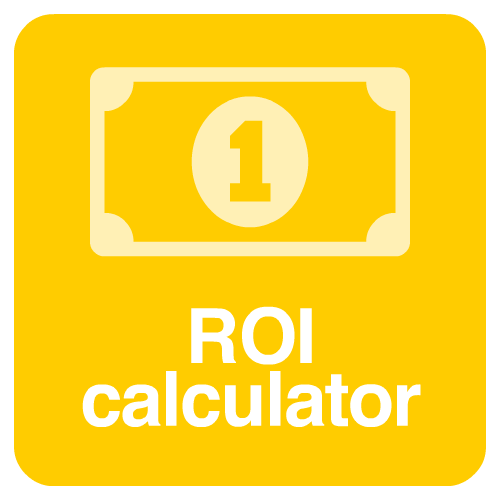Aspect Ratios

# ROI calculator

## return on investmentROI calculator is basically our tool for calculating the return on investment. We developed this calculator simply to be the easiest to use, but with all the features you are looking for. With it you can calculate investments that you have already made or will still make.

### Understand how our ROI Calculator works

You can calculate your return on investment by basically defining the amount invested and the time the investment lasted, and our tool does it easily. You can calculate ROI for days invested, months and even years, but you can have total return and annual ROI.

To help you make other calculations we are always developing new calculators, such as our Percentage Calculator.

### tips and information

#### What is ROI?

Return on Investment, which is basically known as ROI, is common information used to assess the profit you have made or will still make on an investment. It is very important information before you invest. In something you want to invest,  you can have a clear vision about the return you can expect. But do not think that it is used only for financial investments as you can use it if you want to invest in a company, in asset acquisitions and more.

You can find other ways to calculate the return on an investment, but the ROI method is the most used and therefore the simplest.

#### How to calculate ROI?

You can calculate ROI basically like this:

ROI = (amount earned – amount invested) divided by the amount invested

In short, if the amount invested was \$ 1,000 and the return was \$ 1,500, it is:

ROI = (\$ 1,500 – \$ 1,000) divided by \$ 1,000

In this example above the ROI was 50%